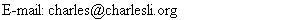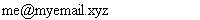## Use image of E-mail to avoid spam mail.

Charles LiCharles' Java Articles | charlesli.org

Everyone knows how irritated spam mails are. Spammer can use robot program to crawl through websites (e.g. find <a href="mailto:me@myemail.xyz">). One very simple way to fight the robot program is to use image files to replace the E-mail address.
Here is a simple program to create an E-mail image

```import javax.imageio.*;
import java.io.*;
import java.awt.image.*;
import java.awt.*;

public class EmailImage {

public static void main(String[] args) {
int width = 200; // numbers by trial and error
int height = 20;
String email = "me@myemail.xyz";
String imageName = "xyz.png";

BufferedImage bi = new BufferedImage(width, height, BufferedImage.TYPE_INT_RGB);
Graphics2D g2 = bi.createGraphics();
// setbackgroud color
Color background = Color.white;
g2.setColor(background);
g2.fillRect(0,0, width, height);

g2.setColor(Color.black);
g2.setFont(new Font("Serif", Font.PLAIN, 16));
int x = 2, y = height - 5; // (x,y) coordinate,
// the numbers are figured out by trial and error
g2.drawString(email, x ,y);

File f = new File(imageName);
try {
// png is an image format (like gif or jpg)
ImageIO.write(bi, "png", f);
} catch (IOException ex) {
ex.printStackTrace();
}
}
}
```

After you run this program you will get the following image

`xyz.png`Here is another program. You don't have to figure out the height, width etc. The program will determine the bound of the E-mail at the run time.

```import java.awt.geom.Rectangle2D;
import javax.imageio.*;
import java.io.*;
import java.awt.image.*;
import java.awt.*;

public class EmailImage2 {

public static void main(String[] args) {
// the number here doesn't really matter
int width = 500, height = 500, x = 0, y = 0;

String email = "me@myemail.xyz"; // your E-mail
String imageName = "xyz.png"; // output picture
// Font font = new Font("Serif", Font.PLAIN, 16); // font that you use
Font font = new Font("impact", Font.PLAIN, 16);

// create a graphics, only used to create FontMetrics
// FontMetrics is used to find out the height, width of the String
// when you draw the String on the graphics.
BufferedImage bi = new BufferedImage(width, height, BufferedImage.TYPE_INT_RGB);
Graphics2D g2 = bi.createGraphics();
FontMetrics fm = g2.getFontMetrics(font);
Rectangle2D rect= fm.getStringBounds(email, g2);
height = (int)rect.getHeight();
width = (int)rect.getWidth();
x = 0;
y = - (int)(rect.getY());

// now we get the real height and width of the E-mail
bi = new BufferedImage(width, height, BufferedImage.TYPE_INT_RGB);
g2 = bi.createGraphics();

// setbackgroud color
Color background = Color.white;
g2.setColor(background);
g2.fillRect(0,0, width, height);

g2.setColor(Color.black);
g2.setFont(font);
g2.drawString(email, x ,y);

File f = new File(imageName);
try {
// png is an image format (like gif or jpg)
ImageIO.write(bi, "png", f);
} catch (IOException ex) {
ex.printStackTrace();
}
}
}

```

Charles' Java Articles | charlesli.org |
First posted: 9/22/2005
Last modifed: 9/22/2005